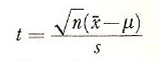A# student's t-distribution

In statistics, the student's t-distribution is a way of testing how closely a model of population corresponds to the results of sampling. t is given bywhere n is the number of items in the sample, s the sample standard deviation, x-bar the sample mean, and μ the mean of the normal distribution, which is one's model.